# How to Check/Test if Sheets Exist in Excel?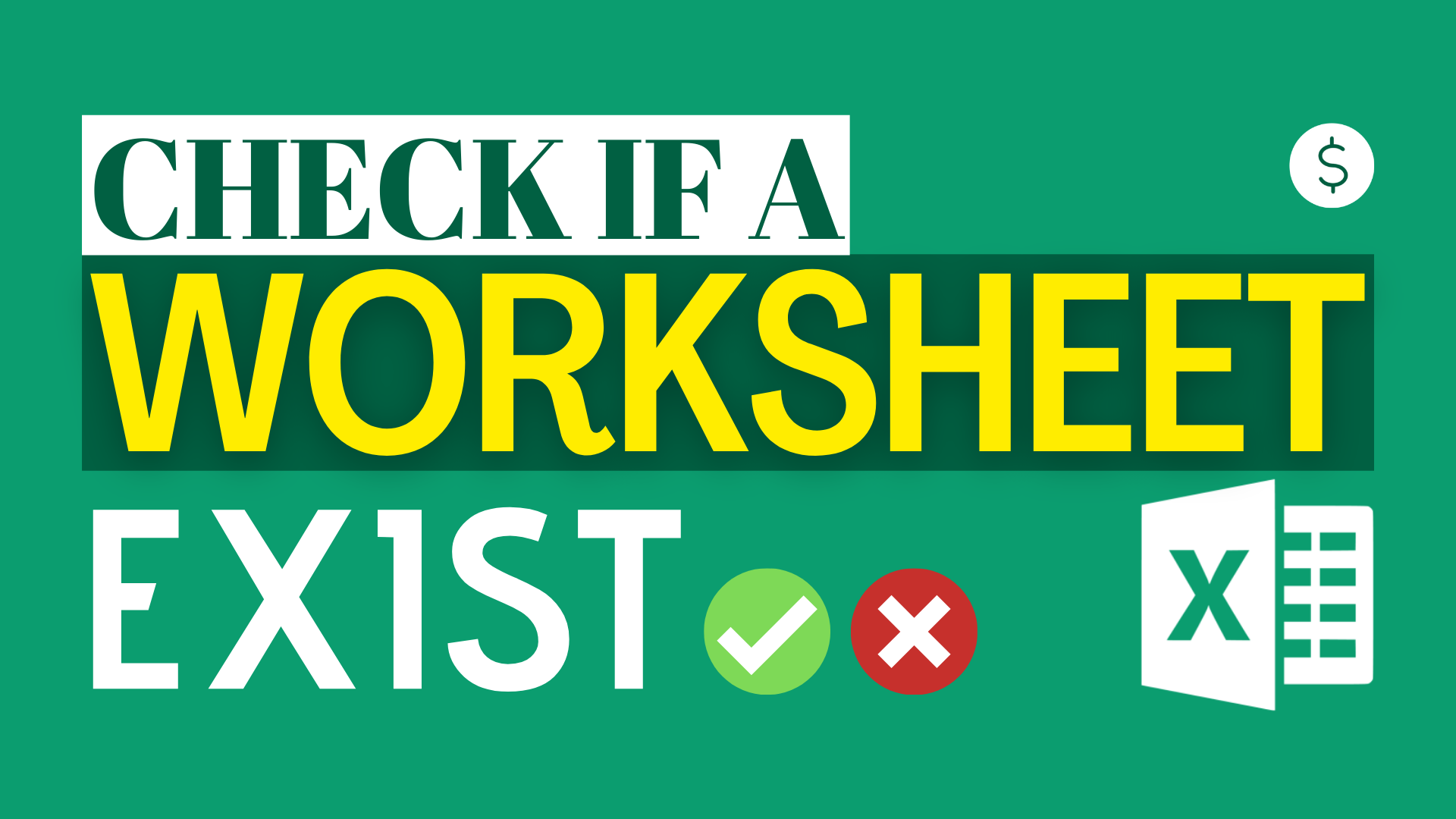## Background

Sometimes we would like to check if sheets exist in Excel. This is easy when you only have a few worksheets.

However, if you have hundreds of sheet, it is not as easy. Even if you have the time to check the Excel tab one by one, you may still make mistakes.

Do you have the following questions?

• How to determine if a sheet exists in a workbook?
• How can I tell if sheet a exists in a workbook?
• How to check whether a sheet exists or not?
• What is the VBA code to test if a sheet exists or not?

In this article, I will show you how to check or test if sheets exist in Excel using two ways including a formula and VBA.

## Option 1 By Formula

Formula of cell B2

`=ISREF(INDIRECT("'"&\$A2&"'"&"!A2"))`

Formula of cell B3

`=ISREF(INDIRECT("'"&\$A3&"'"&"!A3"))`

Formula of cell B4

`=ISREF(INDIRECT("'"&\$A4&"'"&"!A4"))`

Formula of cell B5

`=ISREF(INDIRECT("'"&\$A5&"'"&"!A5"))`

INDIRECT function

Syntax:

`=INDIRECT(ref_text,[a1])`

This function returns the value based on a text string.

For example, INDIRECT(“B2”) returns the value of cell B2.

ISREF function

Syntax:

`=ISREF(value)`

This function checks if a cell contains a valid reference.

If the cell contains a valid reference, it returns “TRUE”. If the cell doesn’t contain a valid reference, it returns “FALSE”.

Explanation

`=ISREF(INDIRECT("'"&\$A2&"'"&"!A2"))`

In this formula, we first use INDIRECT function to return the sheet name, then use ISREF function to determine if the sheet name is a valid reference.

Text highlighted in red are the variables.

`("'"&\$A2&"'"&"!A2")`

This part is to form the cell reference. It becomes (‘2019 Jan’!A2) eventually.

With ISREF function, it becomes ISREF(‘2019 Jan’!A2).

Since (‘2019 Jan’!A2) is valid reference, it will return TRUE.

## Option 2 By VBA

```Option Compare Text
Sub check_if_sheet_exists()
'The script is created by Dollar Excel.
'Subscribe to Dollar Excel newsletter for more free resources like this.

Dim wb As Workbook
Dim ws As Worksheet
Set wb = ActiveWorkbook

testws = InputBox("Input a worksheet name here")
For Each ws In wb.Worksheets
If ws.Name = testws Then
MsgBox "This worksheet exists."
End If
Next
MsgBox "This worksheet does not exist."
End Sub
```

What this VBA code does

This code determines if a worksheet exist. This code is case-insensitive.

Running this VBA, a window will pop up.

If a worksheet reference isn’t valid, this will pop up.

If a worksheet reference isn’t valid, this will pop up.

#### To make the code case-sensitive

Delete the first line of code

`Option Compare Text`

### Other VBA articles

How to Check/Test if Sheets Exist in Excel?

How To List All Worksheets Name In A Workbook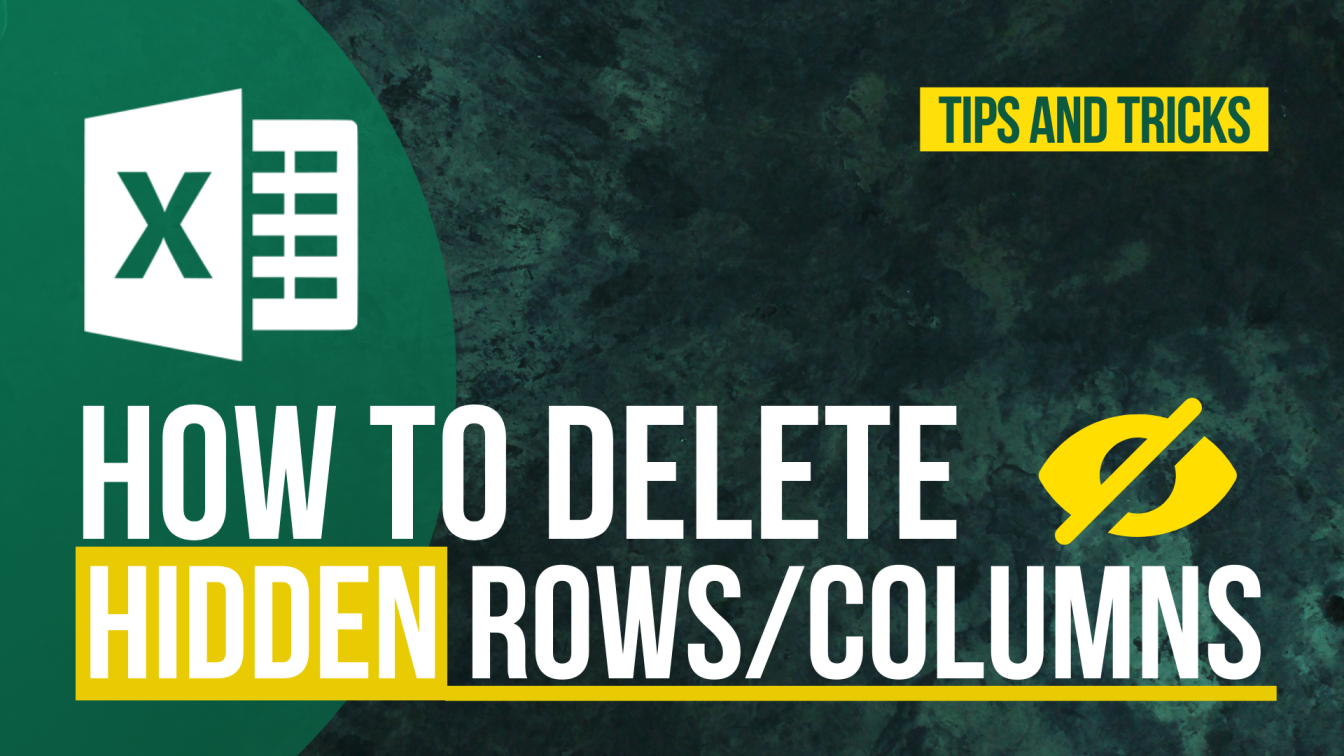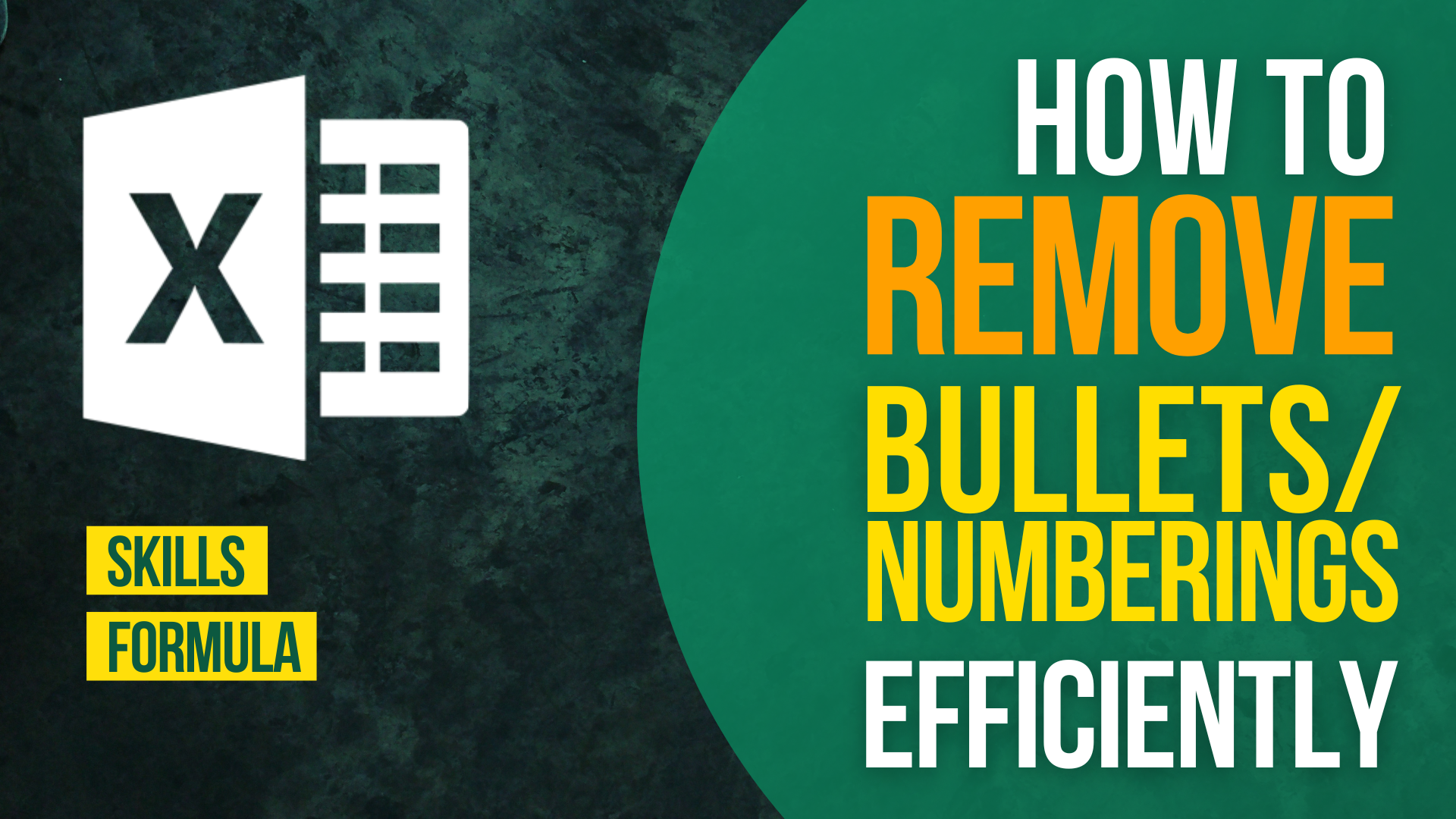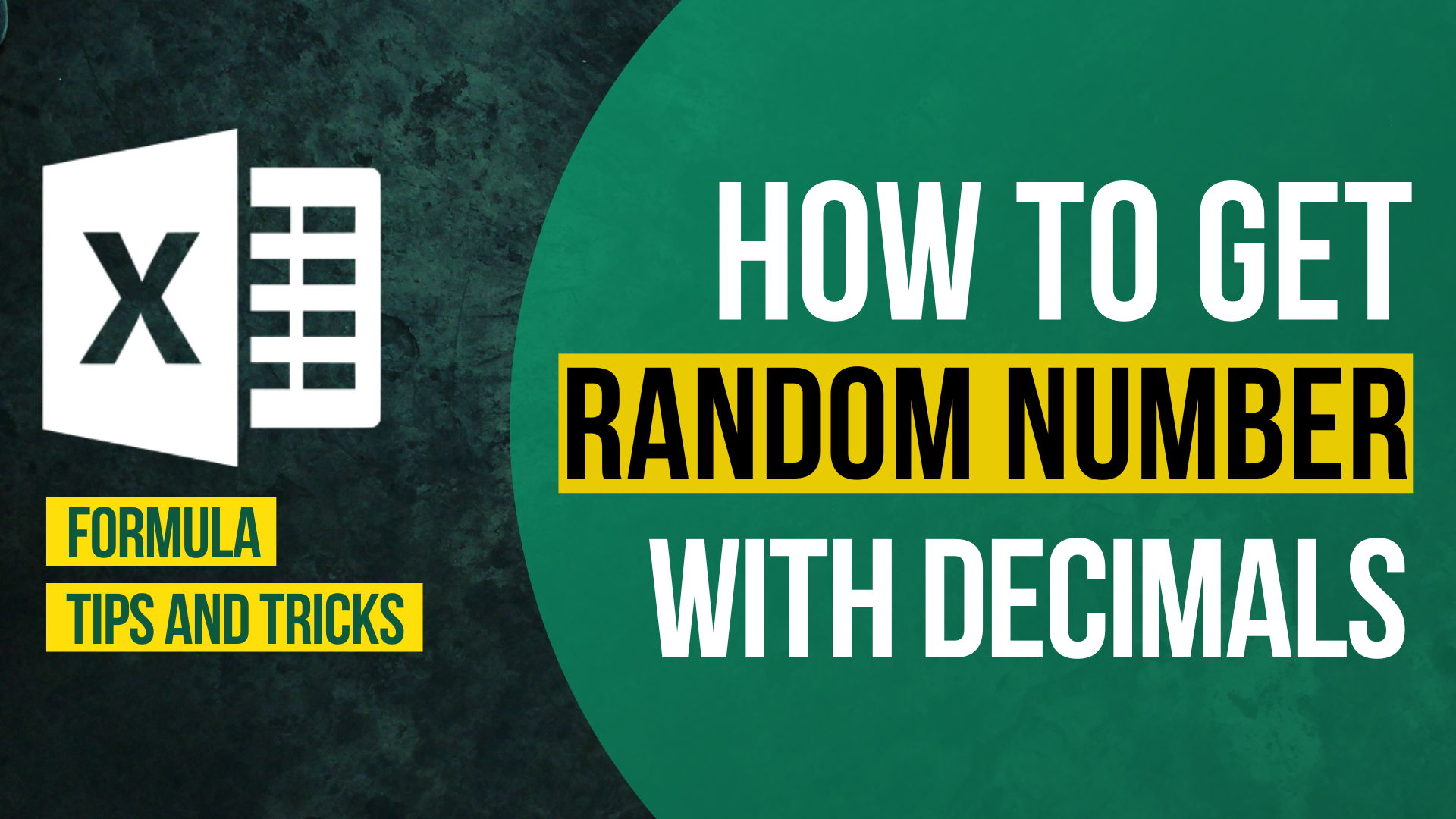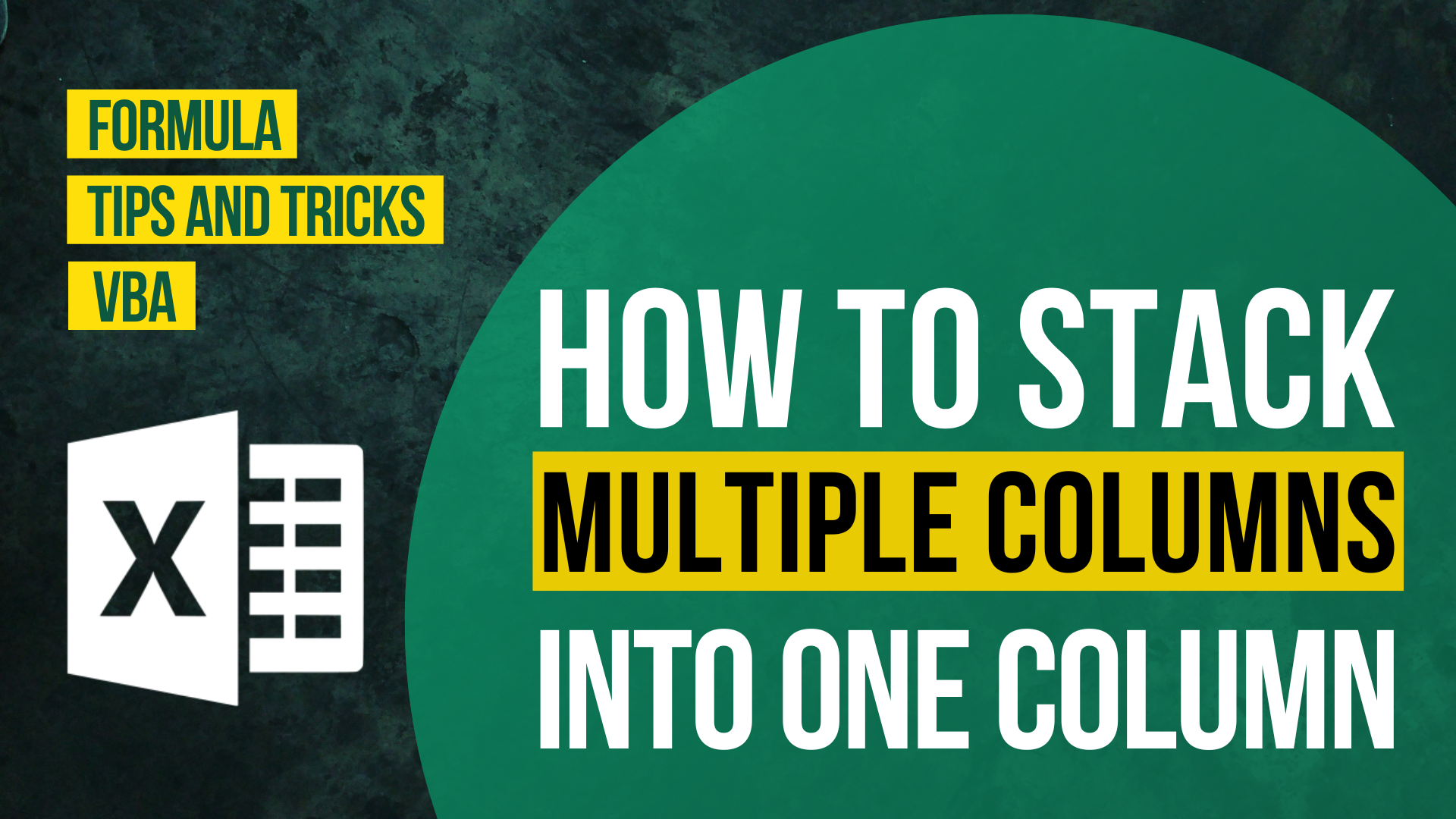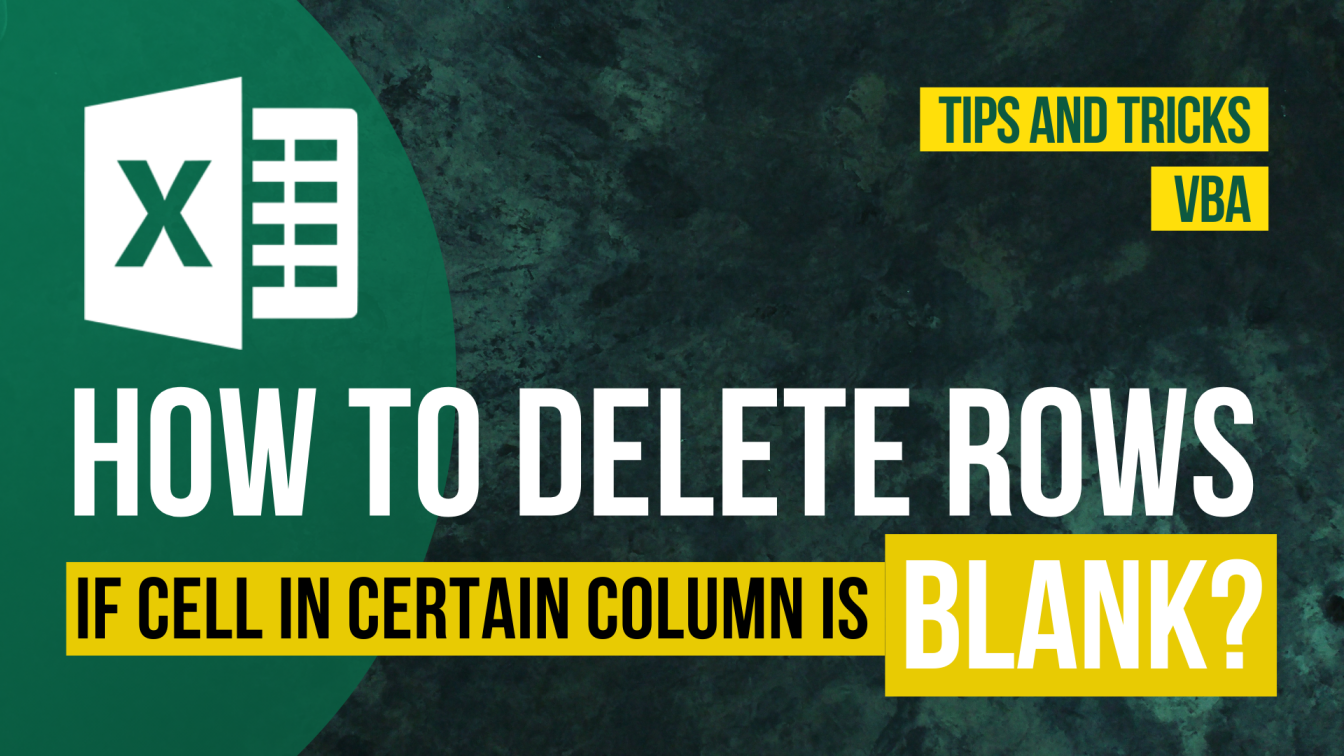How to Input Time Duration in Excel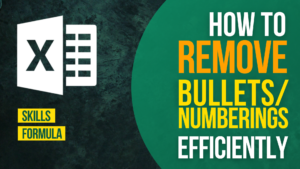How to Remove Bullets/Numberings in Excel

Popular posts